Site search
Web search
 Index Home Departments Teachers Students Parents High School Middle School Elementary School Administration Contact Us SponsorshipArticles Books Videos Tutorials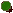Help.comE CalculusCalculus PrimerA Calculus Primer Some graphics reproduced using Print Shop Deluxe, Broderbund Software, Inc. All Rights Reserved used by permission. The Classroom does not claim all descriptions of sites to be their own words. The Classroom  makes no promises or representations about the gadgets on this site as to quality. content or  performance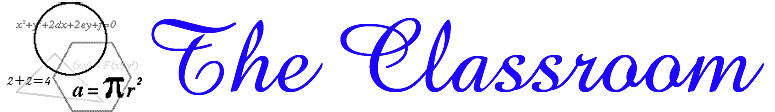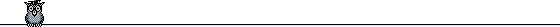Calculus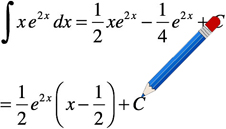Calculus: The study of Mathematical Change. It has two branches Differential  and Integral Calculus.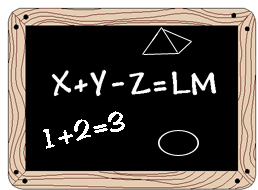Mathematics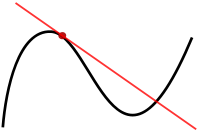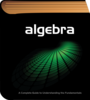Geometry Algebra Trigonometry Pre Algebra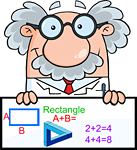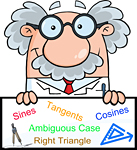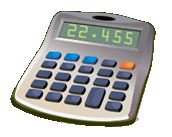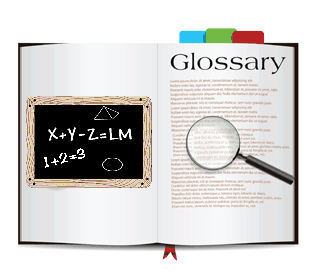Formulas/Tables Laws Calculator Glossary
Differential CalculusModulesApplicationsKhan AcademyWikipediaStudy.comMath Help Boards
Integral CalculusModulesWikipediaKhan AcademyCalculus PrimerPaul's..Math Notes
Calculus CalculatorsIFigureEMath HelpMath PortalSymbolab.comWolfram-ExamplesCalculus-calculator.com
Online ResourcesWikipediaEric DigestAll About CalculusLee Lady Topics in CalculusStudy StackThe Calculus PageThe Calculus PageThe History Of CalculusKhan AcademyEncyclopedia of MathCalculus on the WebCalculus Made Easier